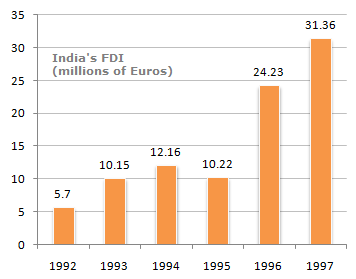# Data Interpretation - Bar Charts - Discussion

The following bar chart shows the trends of foreign direct investments(FDI) into India from all over the world.

Trends of FDI in India5.

What was India's total FDI for the period shown in the figure ?

 [A]. 93.82 [B]. 93.22 [C]. 93.19 [D]. None of these

Explanation:

Total FDI investment in the figure shown is = 5.7 + 10.15 + 12.16 + 10.22 + 24.23 + 31.36 = 93.82 billion.

 Sanjay said: (Mar 19, 2012) As neither any option nor question carries unit billion, so I thought it should be D i.e. none of these. Please explain why answer is A. ?

 Ashu said: (May 28, 2014) its wrong ans write is 93.79

 Imran said: (Jun 20, 2015) If I calculate the total manually I get 93.19 but when calculated with calculator I get 93.82?

 Ron said: (Nov 19, 2015) @Imran. In year 1992, 5.7--> add this as 5.70.

 Sri said: (Jul 26, 2018) Yeah, it is the correct answer place it as 5.70. Agree @Ron.